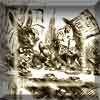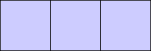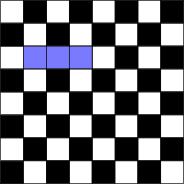#### You may also like### A Problem of Time

Consider a watch face which has identical hands and identical marks for the hours. It is opposite to a mirror. When is the time as read direct and in the mirror exactly the same between 6 and 7?### Eight Dominoes

Using the 8 dominoes make a square where each of the columns and rows adds up to 8### Holly

The ten arcs forming the edges of the "holly leaf" are all arcs of circles of radius 1 cm. Find the length of the perimeter of the holly leaf and the area of its surface.

# Trominoes

##### Age 11 to 16Challenge Level

A tromino is a $3 \times 1$ block:What sized rectangles may be made using trominoes? You can print off and cut out trominoes from this sheet . Alternatively, if you have a set of the game Jenga, then use the blocks as trominoes (but check they are $3 \times 1$ first)Can you cover $63$ squares of an $8\times 8$ chessboard using trominoes? (Why can't you cover $64$?). If so, which square remains uncovered, and are there other arrangements of the trominoes which would lead to a different square being left uncovered?

Think of some other squares which cannot be covered with trominoes. Can you "almost" cover them, leaving only one hole? When can an $n \times n$ square be covered or "almost" covered?